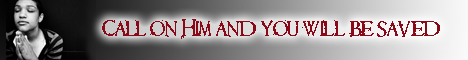Note:  Do not rely on this information. It is very old.

# Sine

Sine is a tdgonometrical ratio by means of which an angle can be measured. If from any point B in one line bounding an angle A, a perpendicular B C be dropped on to the other line, then the ratio B C/ A C is called the sine of the angle A. With A. as centre and radius A B, we describe the arc B D of a circle, then the old definition of sine referred to it as a function of the arc B D, while the line B C - not the ratio used above - was said to be the sine of B D. The length, B C, varied with the radius of the circle, but the modern definition overcomes this difficulty, for sine of the angle A = sine of the arc / radius of the circle